# Finding Area Using Integrals

To evaluate a definite integral, we find the antiderivative, evaluate it at the indicated bounds, and then take the difference.

This post is part of a series.

In the last post, we learned how to evaluate integrals of the form $\int f(x) \, dx$, which are also known as indefinite integrals.

In this chapter, we shall be concerned with definite integrals, which have bounds of integration. The definite integral $\int_a^b f(x) \, dx$ is evaluated by first finding the antiderivative $F(x) = \int f(x) \, dx$, and then computing the difference between the values of the antiderivative at the indicated bounds.

\begin{align*} \int_a^b f(x) \, dx = F(b) - F(a) \end{align*}

## Derivation

Subtracting at the bounds yields the area between the x-axis and the function $f(x)$, between the bounds $x=a$ and $x=b$. To see why, first consider that $F(b)-F(a)$ is the sum of infinitely many, infinitely small changes in $F$, one for each value of $x$. At each value $x$, the function has slope $F’(x)$, so if it travels an infinitesimal $dx$ units to the right, then it also travels an infinitesimal $F’(x) \, dx$ units up.

\begin{align*} F(b)-F(a) &= \sum\limits_{x \in [a,b]} F'(x)\, dx \end{align*}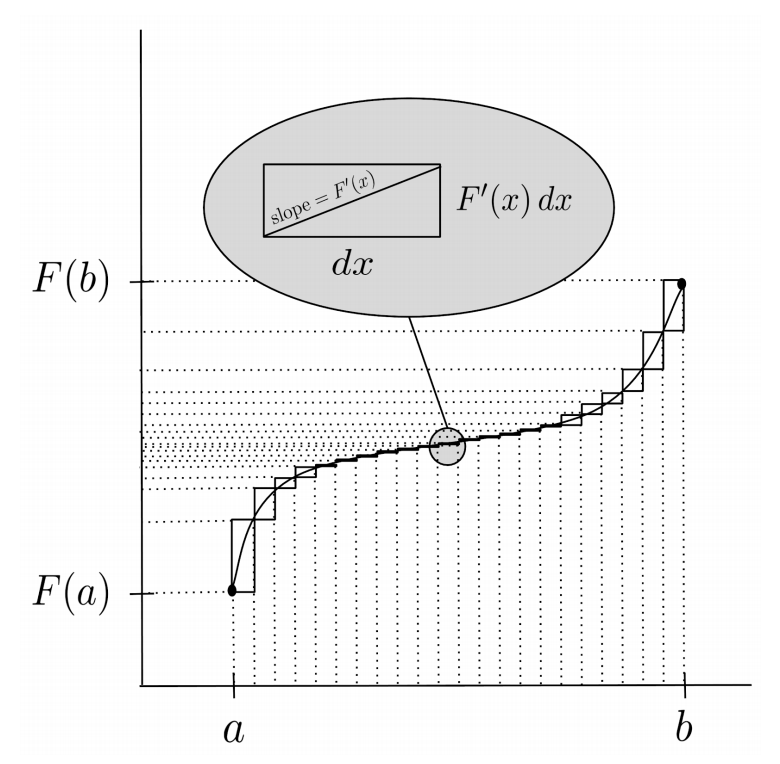This is true even if the function doubles back on itself, because the upward and downward displacements cancel each other out.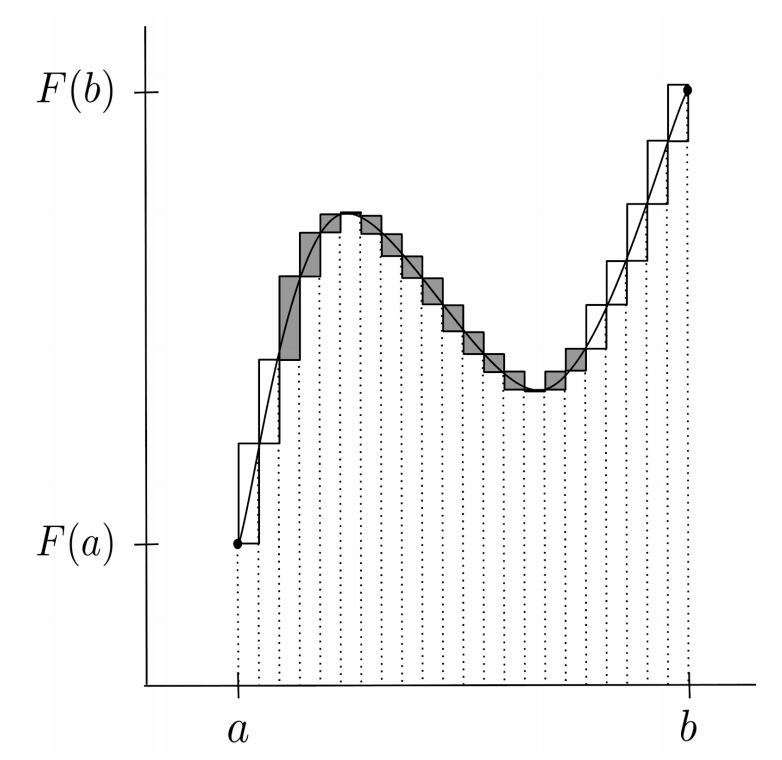Because $F(x) = \int f(x) \, dx$, we have $F’(x)=f(x)$, so we can write the sum in terms of $f$.

\begin{align*}F(b)-F(a) &= \sum\limits_{x \in [a,b]} F'(x)\, dx \\ &= \sum\limits_{x \in [a,b]} f(x) \, d x \end{align*}

Each term in the sum then corresponds to the area of a rectangle of width $dx$ and height $f(x)$, and all the rectangles together make up the area between the x-axis and the graph of $f$.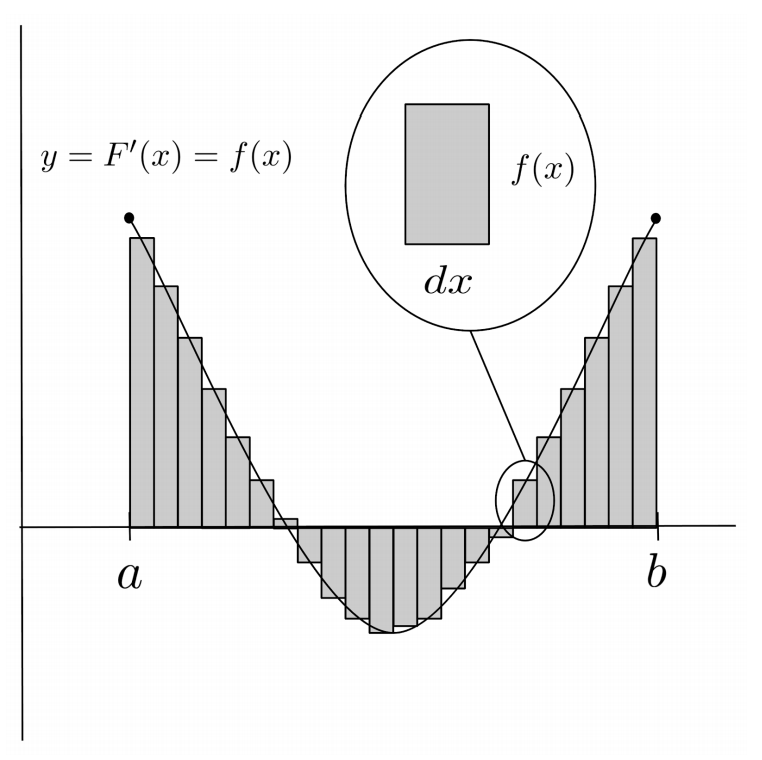## Sanity Check

Below is an example of evaluating a simple definite integral.

\begin{align*} \int_0^1 2x \, dx &= \left[ x^2 \right]_0^1 \\ &= 1^2 - 0^2 \\ &= 1-0 \\ &= 1 \end{align*}

We can verify that this result represents the area between the x-axis and the function $f(x)=2x$ between the bounds $x=0$ and $x=1$, because this region is just a triangle.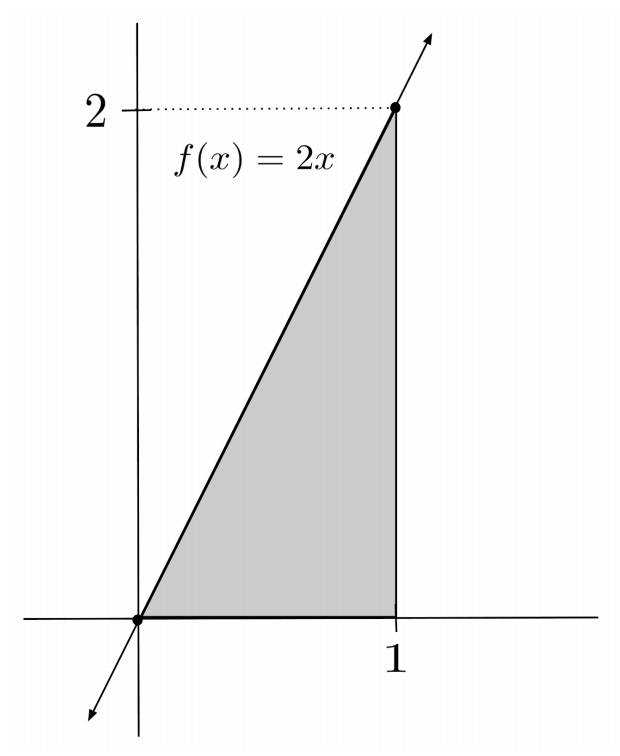\begin{align*} \mbox{area of triangle}& = \frac{1}{2}\mbox{(base)}\mbox{(height)} \\ &= \frac{1}{2}(1)(2) \\ &= 1 \end{align*}

The results match up!

## Demonstration

Now, let’s compute the area between the x-axis and the parabola $f(x)=2x^2$, between the same bounds $x=0$ and $x=1$. The parabola dips a little lower than the triangle which we found has area $1$, so we should expect a result a little smaller than $1$.\begin{align*} \int_0^1 2x^2 \, dx &= \left[ \frac{2}{3} x^3 \right]_0^1 \\ &= \frac{2}{3}(1)^3 - \frac{2}{3}(0)^3 \\ &= \frac{2}{3} - 0 \\ &= \frac{2}{3} \end{align*}

The area is $\frac{2}{3}$, which is indeed slightly smaller than $1$, so it matches up with our expectations.

## Negative Area

When a function dips below the x-axis, the area below the x-axis is counted as negative area.

For example, if we integrate the function $f(x)=-2x$ between the bounds $x=0$ and $x=1$, we get a result of $-1$. This is the same triangle as before, but flipped over the x-axis.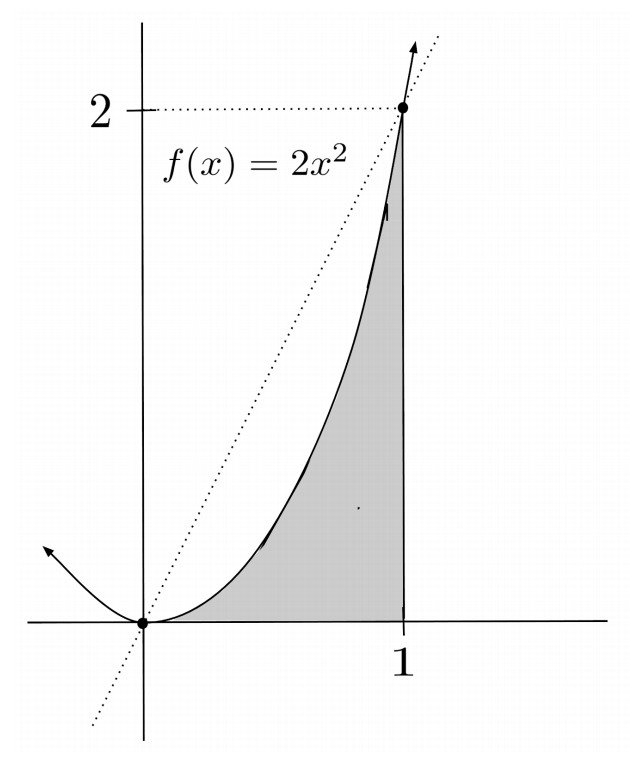\begin{align*} \int_0^1 -2x \, dx &= \left[ -x^2 \right]_0^1 \\ &= -(1)^2 - (-(0)^2) \\ &= -1+0 \\ &= -1 \end{align*}

As a consequence of negative area, for a region that has the same amount of area above the x-axis as below the x-axis, the integral will evaluate to $0$.

For example, the function $f(x)=2x$ integrates to zero on the interval from $x=-1$ to $x=1$ because its two triangles above and below the x-axis cancel each other out.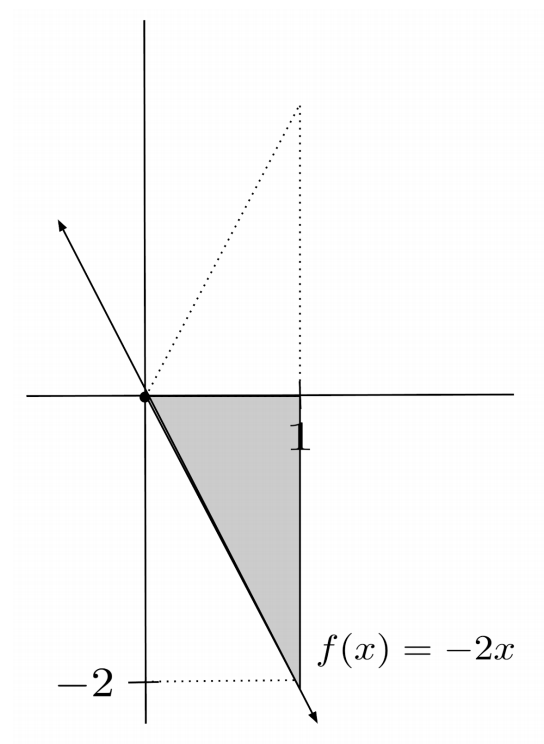\begin{align*} \int_{-1}^1 2x \, dx &= \left[ x^2 \right]_{-1}^1 \\ &= 1^2 - (-1)^2 \\ &= 1-1 \\ &= 0 \end{align*}

## Area Between Two Functions

In addition to finding the area under a single function, integrals can also be used to find the area between two functions.

If we have two functions $f(x)$ and $g(x)$ with $f(x) \geq g(x)$ on the interval $[a,b]$, then the area between the functions on the interval $[a,b]$ is given by the integral of the difference:

\begin{align*} \mbox{area between } f \mbox{ and } g = \int_a^b f(x)-g(x) \, dx \end{align*}

One way to interpret the integral above is to see it as the difference of two separate integrals, the integral of $f$ minus the integral of $g$. Then the area between $f$ and $g$ is the area under $f$ minus the overlapping area under $g$, which leaves only the area between $f$ and $g$.

\begin{align*} \int_a^b f(x)-g(x) \, dx &= \int_a^b f(x) \, dx - \int_a^b g(x) \, dx \\ &= (\mbox{area under } f) - (\mbox{area under } g) \\ &= \mbox{area between } f \mbox{ and } g \end{align*}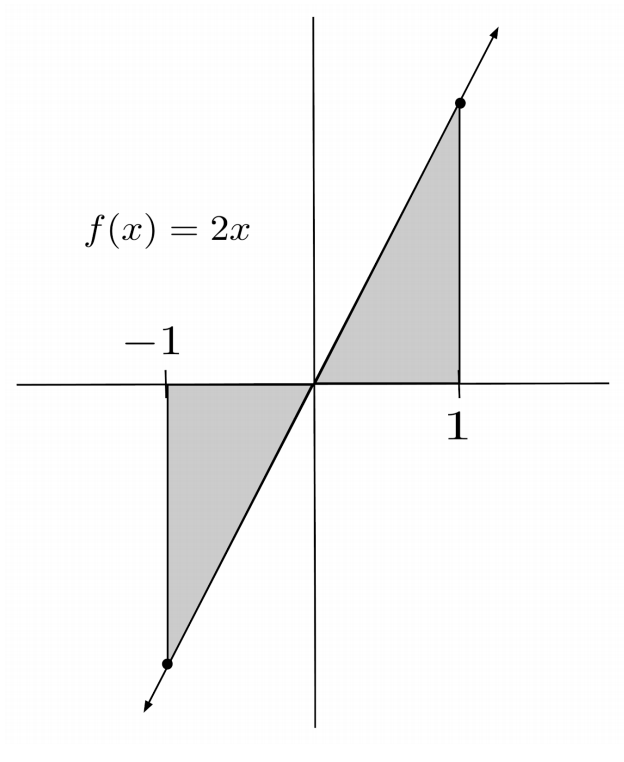Another way to interpret the integral is to see it as integrating the height from $g$ to $f$. In this case, we are defining a height function $h(x)=f(x)-g(x)$ and breaking the region between the functions into infinitesimally small rectangles, each rectangle having height $h(x)$ and infinitesimal width $dx$.For example, to find the area between $y=3x+1$ and $y=2x^2-x$ on the interval $[1,2]$, we first need to identify which function is the higher one on this interval. We can do this by sketching graphs of the functions.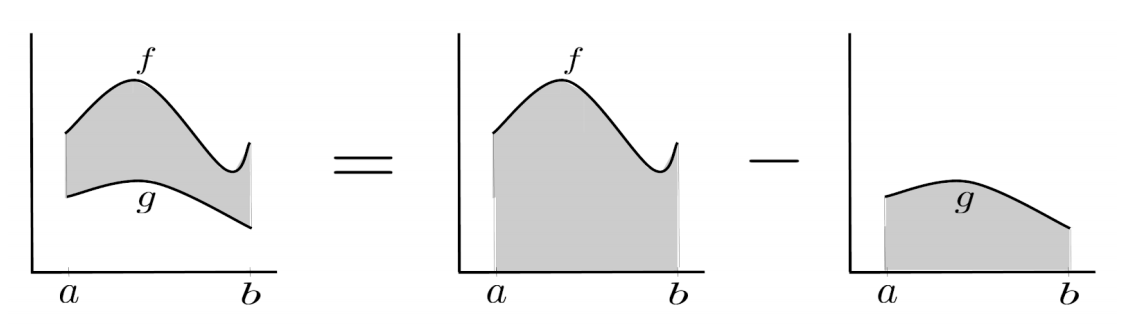We see that $y=3x+1$ is the higher function and $y=2x^2-x$ is the lower function. The integral is the higher function $3x+1$ minus the lower function $2x^2-x$, over the interval $[1,2]$.

\begin{align*} &\int_1^2 (3x+1)-(2x^2-x) \, dx \\ &= \int_1^2 3x+1-2x^2+x \, dx \\ &= \int_1^2 -2x^2 + 4x + 1 \, dx \\ &= \left[ -\frac{2}{3}x^3 + 2x^2 + x \right]_1^2 \\ &= \left[ -\frac{2}{3}(2)^3 + 2(2)^2 + (2) \right] - \left[ -\frac{2}{3}(1)^3 + 2(1)^2 + (1) \right] \\ &= \left[ -\frac{16}{3}+ 8 + 2 \right] - \left[ -\frac{2}{3} + 2 + 1 \right] \\ &= \left[ \frac{14}{3} \right] - \left[ \frac{7}{3} \right] \\ &= \frac{7}{3} \end{align*}

So, the area between the functions $y=3x+1$ and $y=2x^2-x$ on the interval $[1,2]$ is $\frac{7}{3}$.

## Area Between Two Functions that Intersect

Sometimes, two functions will cross on an interval, and each will take its turn being the higher function. For example, the functions $y=x$ and $y=x^2$ cross twice on the interval $[-1,3]$. The points of intersection are obtained by setting the functions equal to each other and solving:

\begin{align*} x &= x^2 \\ 0 &= x^2-x \\ 0 &= x(x-1) \\ x &= 0,1 \end{align*}

On $[-1,0]$ the higher function is $y=x^2$, on $[0,1]$ the higher function is $y=x$, and on $[1,3]$ the higher function is $y=x^2$. To find the total area bounded between the functions, we integrate the higher function minus the lower function on each interval and add the results together.

\begin{align*} &\int_{-1}^0 x^2-x \, dx + \int_0^1 x-x^2 \, dx + \int_1^3 x^2-x \, dx \\ &= \left[ \frac{1}{3}x^3 - \frac{1}{2}x^2 \right]_{-1}^0 + \left[ \frac{1}{2}x^2 - \frac{1}{3}x^3 \right]_{0}^1 + \left[ \frac{1}{3}x^3 - \frac{1}{2}x^2 \right]_{1}^3 \\ &= \left[ - \left( -\frac{1}{3} - \frac{1}{2} \right) \right] + \left[ \frac{1}{2} - \frac{1}{3} \right] + \left[ \left( 9-\frac{9}{2} \right) - \left( \frac{1}{3}-\frac{1}{2} \right) \right] \\ &= \frac{5}{6} + \frac{1}{6} + \frac{28}{6} \\ &= \frac{17}{3} \end{align*}

To perform the computation faster, we can ignore which function is higher vs lower provided we take the absolute value of each integral before adding them together. Even if we end up “incorrectly” computing the lower function minus the higher function in some integral, the result will still represent area – it will just be negative area, so we can correct it by making it positive.

If we treat $y=x^2$ as the higher function on all intervals but take the absolute value of the integrals before adding them, we reach the same result as before.

\begin{align*} &\left| \int_{-1}^0 x^2-x \, dx \right| + \left| \int_0^1 x^2-x \, dx \right| + \left| \int_1^3 x^2-x \, dx \right| \\ &= \left| \left[ \frac{1}{3}x^3 - \frac{1}{2}x^2 \right]_{-1}^0 \right| + \left| \left[ \frac{1}{3}x^3 - \frac{1}{2}x^2 \right]_{0}^1 \right| + \left| \left[ \frac{1}{3}x^3 - \frac{1}{2}x^2 \right]_{1}^3 \right| \\ &= \left| \frac{5}{6} \right| + \left| -\frac{1}{6} \right| + \left| \frac{28}{6} \right| \\ &= \frac{5}{6} + \frac{1}{6} + \frac{28}{6} \\ &= \frac{17}{3} \end{align*}

Likewise, if we treat $y=x$ as the higher function on all intervals but take the absolute value of the integrals before adding them, we reach the same result as before.

\begin{align*} &\left| \int_{-1}^0 x-x^2 \, dx \right| + \left| \int_0^1 x-x^2 \, dx \right| + \left| \int_1^3 x-x^2 \, dx \right| \\ &= \left| \left[ \frac{1}{2}x^2 - \frac{1}{3}x^3 \right]_{-1}^0 \right| + \left| \left[ \frac{1}{2}x^2 - \frac{1}{3}x^3 \right]_{0}^1 \right| + \left| \left[ \frac{1}{2}x^2 - \frac{1}{3}x^3 \right]_{1}^3 \right| \\ &= \left| -\frac{5}{6} \right| + \left| \frac{1}{6} \right| + \left| -\frac{28}{6} \right| \\ &= \frac{5}{6} + \frac{1}{6} + \frac{28}{6} \\ &= \frac{17}{3} \end{align*}

## Exercises

Find the net (signed) area below each function on the given interval. (You can view the solution by clicking on the problem.)

\begin{align*}1) \hspace{.5cm} &y = 12x^3 + x \\ &x \in [-1,2] \end{align*}
Solution:
\begin{align*} \frac{93}{2} \end{align*}

\begin{align*}2) \hspace{.5cm} &y = \sin x - \sec^2 x \\ &x \in \left[ 0, \frac{\pi}{3} \right] \end{align*}
Solution:
\begin{align*} \frac{1}{2} - \sqrt{3} \end{align*}

\begin{align*}3) \hspace{.5cm} &y = 3e^{5x} \\ &x \in [-2,0] \end{align*}
Solution:
\begin{align*} \frac{3}{5} \left( 1-e^{-10} \right) \end{align*}

\begin{align*}4) \hspace{.5cm} &y = \sqrt{x} + \sin x \\ &x \in [\pi, 9\pi] \end{align*}
Solution:
\begin{align*} \frac{52}{3} \pi^\frac{3}{2} \end{align*}

Find the area between the two functions on the given interval. (You can view the solution by clicking on the problem.)

\begin{align*}5) \hspace{.5cm} &y = 2^x \\ &y=e^x \\ &x \in [0,1] \end{align*}
Solution:
\begin{align*} e-1-\frac{1}{\ln 2} \end{align*}

\begin{align*}6) \hspace{.5cm} &y = x-1 \\ &y=x^2 \\ &x \in [-2,2]\end{align*}
Solution:
\begin{align*} \frac{28}{3} \end{align*}

\begin{align*}7) \hspace{.5cm} &y = \sqrt{x} \\ &y= \ln x \\ &x \in [e,e^2] \end{align*}
Solution:
\begin{align*} \frac{1}{3} \left( 2e^3 - 3e^2 - 2e^\frac{3}{2} \right) \end{align*}

\begin{align*}8) \hspace{.5cm} &y = \cos x \\ &y= 4-x^2 \\ &x \in \left[ -\frac{\pi}{2}, \frac{\pi}{2} \right] \end{align*}
Solution:
\begin{align*} 4\pi - 2 - \frac{\pi^3}{12} \end{align*}

\begin{align*}9) \hspace{.5cm} &y = x+1 \\ &y=x^2-1 \\ &x \in [1,3] \end{align*}
Solution:
\begin{align*} 3 \end{align*}

\begin{align*}10) \hspace{.5cm} &y = \sin x \\ &y= \cos x \\ &x \in [0,\pi] \end{align*}
Solution:
\begin{align*} 2\sqrt{2} \end{align*}

\begin{align*}11) \hspace{.5cm} &y = x^2 \\ &y= \sqrt{x} \\ &x \in [-1,8] \end{align*}
Solution:
\begin{align*} \frac{319}{2} \end{align*}

\begin{align*}12) \hspace{.5cm} &y = 1-x^2 \\ &y=x^2-1 \\ &x \in [-2,2] \end{align*}
Solution:
\begin{align*} 8 \end{align*}

This post is part of a series.

Tags: